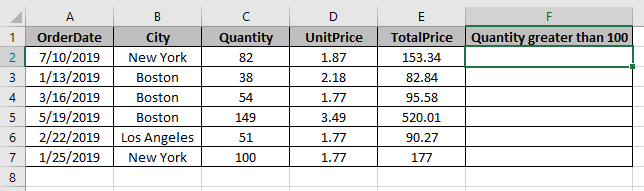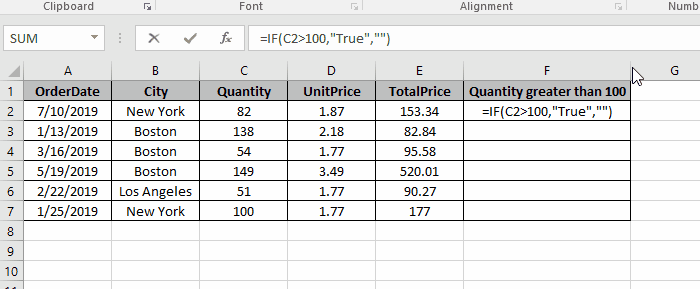# How to check If Cell is greater than given value in Excel

In this article, we will learn about how to return output IF cell is greater than some value in Excel.

We wish to find the function which first compares the cell value with a specific value and then returns output based on the comparison. Simple, we need a Logic_test function. Excel IF function can do this task easily.

Syntax:

=IF( cell_value > value , “value_if_true”, “value_if_false”)

Let’s understand this function using it an example.

Here we have data. We need to see if the quantity > 100, returns “True” or the empty string (“ ”).Use the Formula

=IF(C2>100, “True”, “ ”)

Explanation
The function checks all the value in C2 column and returns “True” if condition stands True else an empty string(“ ”).As you can see, the values in C column which are less than or equals to 100 returns empty string and which are greater than 100 returns True.

Hope you understood how to return output IF cell is greater than some value in Excel. Explore more articles on Excel function here. Please feel free to state your query or feedback for the above article.

Related Articles:

How to Sum If Greater Than 0 in Excel

How to Sum if date is greater than given date in Excel

Retrieving the First Value in a List that is Greater / Smaller than a Specified Value

How to Count cells if less than value in Excel

How to SUM if value is less than given value in Excel

Popular Articles:

50 Excel Shortcuts to Increase Your Productivity

The VLOOKUP Function in Excel

COUNTIF in Excel 2016

How to Use SUMIF Function in Excel

Terms and Conditions of use

The applications/code on this site are distributed as is and without warranties or liability. In no event shall the owner of the copyrights, or the authors of the applications/code be liable for any loss of profit, any problems or any damage resulting from the use or evaluation of the applications/code.